Wind power is a free and clean alternative to traditional fossil fuels.

The theoretical power generated by wind turbines can be easily calculated with an equation. However, to achieve a more realistic solution, taking into account experimental factors outside the ideal modeling, we can reach a Machine Learning solution.

Using actual data from a wind turbine field and a neural network, we will generate a model that can give us all the information we need about how these wind turbines generate power.

### Contents

This example is solved with Neural DesignerYou can use the free trial to understand how the solution is achieved step by step.

## 1. Application type

The variable to be predicted is continuous (power). Therefore, this is an approximation project.

The basic goal here is to model the energy production as a function of the wind speed.

## 2. Data set

The first step is to prepare the data set, which is the source of information for the approximation problem.

It is composed of:

• Data source.
• Variables.
• Instances.

The file wind-turbine.csv contains the data for this example.

Here the number of variables (columns) is 2, and the number of instances (rows) is 48007.

We have the following variables for this analysis:

• wind-speed, the wind speed at the hub height of the turbine, in meters per second.
• power, the power generated by the turbine for that moment, in kilowatts.

Our target variable will be the last one, power.

The instances are divided into training, selection, and testing subsets. They represent 60%, 20%, and 20% of the original instances, respectively, and are split at random.

Calculating the data distributions helps us check for the correctness of the available information and detect anomalies. The following chart shows the histogram for the power-generated variable: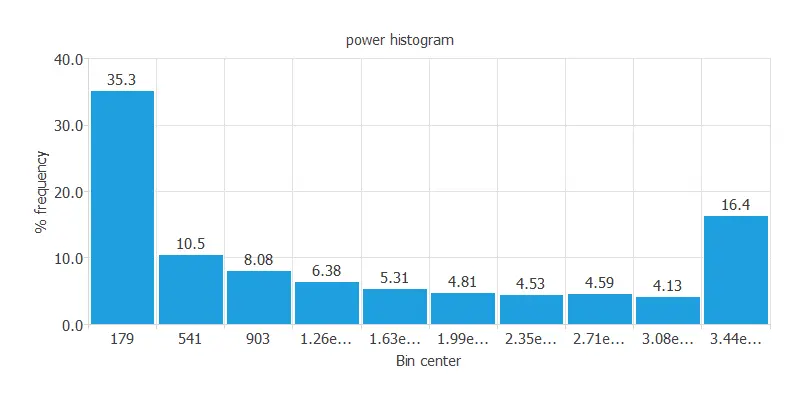It is also interesting to look for dependencies between input and target variables. To do that, we can calculate an inputs-targets correlations table.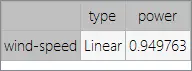As was expected, wind speed is highly correlated to the energy produced by the turbines.

Next, we plot a scatter chart.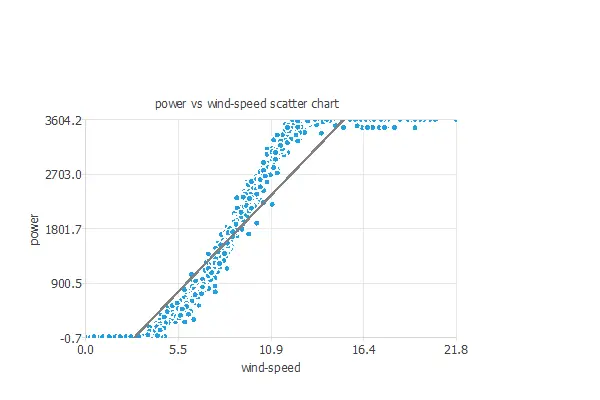We can see here how the power generated, when a threshold wind level is surpassed, increases linearly until an upper bound, where, no matter how superior the wind speed’s magnitude is, the power generated remains the same.

### 3. Neural network

The second step is to build a neural network that represents the approximation function. Approximation problems, it is usually composed by:

• Scaling layer.
• Perceptron layers.
• Unscaling layer.

The neural network has 1 input (wind speed) and 1 output (power).

The scaling layer contains the statistics of the inputs. We use the automatic setting for this layer to accommodate the best scaling technique for our data.

We use 2 perceptron layers here:

The unscaling layer contains the statistics of the outputs. We use the automatic method as before.

The next graph represents the neural network for this example.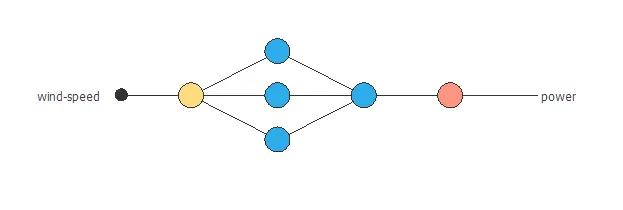## 4. Training strategy

The fourth step is to select an appropriate training strategy. It is composed of two parameters:

• Loss index.
• Optimization algorithm.

The loss index defines what the neural network will learn. It is composed of an error term and a regularization term.

The error term chosen is the normalized squared error. It divides the squared error between the outputs from the neural network and the targets in the data set by its normalization coefficient. If the normalized squared error has a value of 1, then the neural network is predicting the data ‘in the mean’, while a value of zero means a perfect prediction of the data. This error term does not have any parameters to set.

The regularization term is the L2 regularization. It is applied to control the complexity of the neural network by reducing the value of the parameters. We use a weak weight for this regularization term.

The optimization algorithm is in charge of searching for the neural network parameters that minimize the loss index. Here we chose the quasi-Newton method as an optimization algorithm.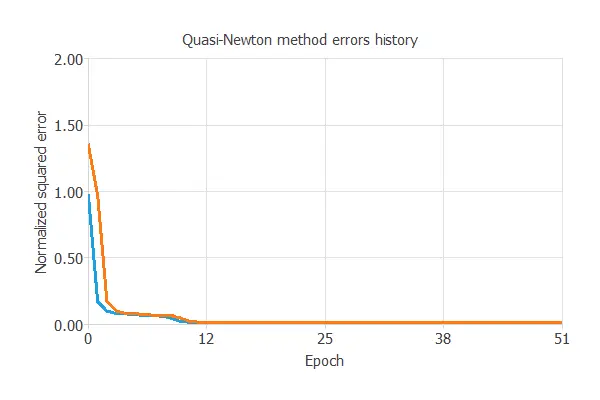The following chart shows how the training (blue) and selection (orange) errors decrease with the epochs during the training process. The final values are training error = 0.00857 NSE and selection error = 0.00869 NSE, respectively.

## 5. Model selection

Model selection algorithms are used to improve the generalization performance of the neural network. As the selection error that we have achieved so far is minimal (0.00869 NSE), this algorithm is not necessary here.

## 6. Testing analysis

The purpose of the testing analysis is to validate the generalization capabilities of the neural network. We use the testing instances in the data set, which have never been used before.

A standard testing method in approximation applications is to perform a linear regression analysis between the predicted and the real pollutant level values.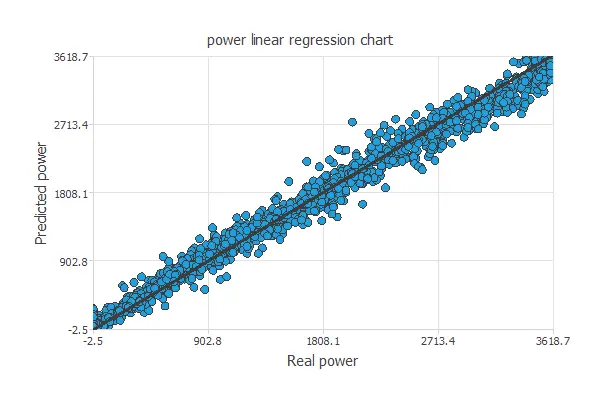For a perfect fit, the correlation coefficient R2 would be 1. As we have R2 = 0.996, the neural network is predicting the testing data very well.

## 7. Model deployment

In the model deployment phase, the neural network is used to predict outputs for inputs that it has never seen.

We can calculate the neural network outputs for a given set of inputs:

• wind-speed: 7.465 metes per second.
• power: 1166.580 kilowatts.

Directional outputs plot the neural network outputs through some reference points.

The reference point for the plot is the one shown next.

• wind-speed: 7.465 metes per second.

We can see here how the wind speed to solar noon affects the power generated: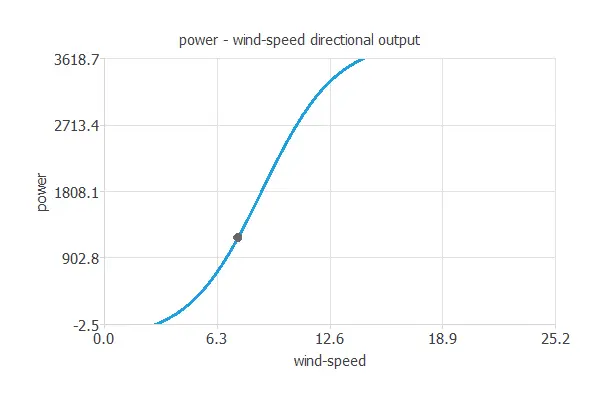This application finds its true meaning when comparing the mean quadratic error of the fabricant’s calculations, using the theoretical turbine power equation (1.95%) versus our model’s error (1.41%), which comes to a 27.69% relative improvement.
Our model performs better because it takes into account the actual empirical data instead of a physical approximation.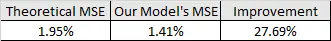The mathematical expression represented by the predictive model is displayed next:

scaled_wind-speed = wind-speed*(1+1)/(25.20599937-(0))-0*(1+1)/(25.20599937-0)-1;
perceptron_layer_output_0 = tanh[ -0.0837533 + (scaled_wind-speed*-0.297207) ];
perceptron_layer_output_1 = tanh[ -0.0199511 + (scaled_wind-speed*0.654869) ];
perceptron_layer_output_2 = tanh[ 0.811123 + (scaled_wind-speed*2.76357) ];
perceptron_layer_output_0 = [ -0.128215 + (perceptron_layer_output_0*0.290953)+ (perceptron_layer_output_1*-0.775739)+ (perceptron_layer_output_2*1.48548) ];
unscaling_layer_output_0 = perceptron_layer_output_0*(3618.72998+2.471410036)/(1+1)-2.471410036+1*(3618.72998+2.471410036)/(1+1);


## 8. Video tutorial

You can watch the step-by-step tutorial video below to help you complete this Machine Learning example